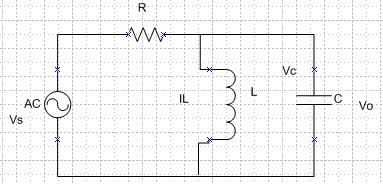# Determine initial conditions of the capacitor's Vc and inductor's Il at t=0

by1990

## Homework Statement

Given the following circuit:[/B]
Where R=C=L=1, with Vs(t) = sin(wt) and complete response Vo(t)=A*sin(wt + π/4).

## Homework Equations

Determine the init. cond. of the capacitor voltage Vc and Inductor current Ic at t = 0. Also, find A and w.[/B]

## The Attempt at a Solution

My thought process told me to find the transfer function in s-domain. I did that by doing laplace transform of Vo and Vs and then divide them. I got
H(s) = A/√2((s+w) / (s^2+w^2))
That's all I got so far. I am lost, some pointers would be greatly appreciated. [/B]

P.S. Is there a proper way to insert equation when creating post?

## Answers and Replies

Staff Emeritus
Hi by1990. http://img96.imageshack.us/img96/5725/red5e5etimes5e5e45e5e25.gif [Broken]

Normally, when you apply drive to a RLC network there is a short duration of transient conditions before the currents and voltages reach steady state sinusoidal values. However, by careful choice of initial conditions (using hidden circuits not relevant to this analysis) you can preset capacitor voltage and inductor current to "prempt" what they would be at that instant in the steady state so that at switch-on the circuit jumps immediately into that steady-state operation without needing to go through that initial exponential transient.

You don't need Laplace.

Last edited by a moderator:
by1990
Hi by1990. http://img96.imageshack.us/img96/5725/red5e5etimes5e5e45e5e25.gif [Broken]

Normally, when you apply drive to a RLC network there is a short duration of transient conditions before the currents and voltages reach steady state sinusoidal values. However, by careful choice of initial conditions (using hidden circuits not relevant to this analysis) you can preset capacitor voltage and inductor current to "prempt" what they would be at that instant in the steady state so that at switch-on the circuit jumps immediately into that steady-state operation without needing to go through that initial exponential transient.

You don't need Laplace.

Hi NasentOxygen!

Thanks for the reply. I understand the idea behind this problem. By looking at the given complete response, I can see that both inductor and capacitor were precharged to certain values so that they cancel out transient response, which presumably in some form of Bep1t + Cep2t. I would assume that the natural response due to initial conditions is in the same form but has right constants and poles which then cancel out the transient response (please correct me if I'm wrong). I'm guessing I can find the init. cond. by doing nodal with the source taken out. But how would one go about to find A and w in the output?

Thanks!

Last edited by a moderator:
Staff Emeritus
You are told what Vo is: Vo(t)=A*sin(wt + π/4)
when Vi(t) = sin(wt)

So you should be able to work out the steady-state instantaneous values of current/voltage for C and L at that moment, i.e., t=0, being whenever the input sinewave crosses the axis heading positive, since the signal is periodic repetitive.

You don't need to calculate the transient, because by using the presets we are evading it. The circuit leaps straight into steady state operation at switch-on, at t=0.

by1990
You are told what Vo is: Vo(t)=A*sin(wt + π/4)
when Vi(t) = sin(wt)

So you should be able to work out the steady-state instantaneous values of current/voltage for C and L at that moment, i.e., t=0, being whenever the input sinewave crosses the axis heading positive, since the signal is periodic repetitive.

You don't need to calculate the transient, because by using the presets we are evading it. The circuit leaps straight into steady state operation at switch-on, at t=0.
I guess I might have made things a little more complicated for myself. Thanks for the clarification!

Homework Helper
Gold Member
So, did you get answers?